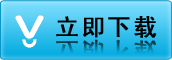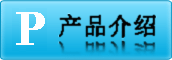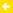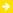三菱(Mitsubishi)FX系列PLCQ系列PLC小A系列PLC大A系列PLCAJ系列CC-Link模块AJ35特殊模块伺服驱动器和伺服电机变频器触摸屏(人机界面)
##### 三菱PLC指令集 三菱PLC指令集

21 SUB 二进制减法运算 √ √ √ √ √
22 MUL 二进制乘法运算 √ √ √ √ √
23 DIV 二进制除法运算 √ √ √ √ √
24 INC 二进制加1运算 √ √ √ √ √
25 DEC 二进制减1运算 √ √ √ √ √
26 WAND 字逻辑与 √ √ √ √ √
27 WOR 字逻辑或 √ √ √ √ √
28 WXOR 字逻辑异或 √ √ √ √ √
29 NEG 求二进制补码 × × × × √

31 ROL 循环左移 × × × × √
32 RCR 带进位右移 × × × × √
33 RCL 带进位左移 × × × × √
34 SFTR 位右移 √ √ √ √ √
35 SFTL 位左移 √ √ √ √ √
36 WSFR 字右移 × × × × √
37 WSFL 字左移 × × × × √
38 SFWR FIFO(先入先出)写入 × × √ √ √
39 SFRD FIFO(先入先出)读出 × × √ √ √

41 DECO 解码 √ √ √ √ √
42 ENCO 编码 √ √ √ √ √
43 SUM 统计ON位数 × × × × √
44 BON 查询位某状态 × × × × √
45 MEAN 求平均值 × × × × √
46 ANS 报警器置位 × × × × √
47 ANR 报警器复位 × × × × √
48 SQR 求平方根 × × × × √
49 FLT 整数与浮点数转换 × × × × √

51 REFF 输入滤波时间调整 × × × × √
52 MTR 矩阵输入 × × √ √ √
53 HSCS 比较置位（速计数用） × √ √ √ √
54 HSCR 比较复位（速计数用） × √ √ √ √
55 HSZ 区间比较（速计数用） × × × × √
56 SPD 脉冲密度 × × √ √ √
57 PLSY 频率脉冲输出 √ √ √ √ √
58 PWM 脉宽调制输出 √ √ √ √ √
59 PLSR 带加减速脉冲输出 × × √ √ √

61 SER 数据查找 × × × × √
62 ABSD 凸轮控制（对式） × × √ √ √
63 INCD 凸轮控制（增量式） × × √ √ √
64 TTMR 示教定时器 × ×   √
65 STMR 特殊定时器 × × × × √
66 ALT 交替输出 √ √ √ √ √
67 RAMP 斜波信号 √ √ √ √ √
68 ROTC 旋转工作台控制 × × × × √
69 SORT 列表数据排序 × × × × √

I/O

70 TKY 10键输入 × × × × √
71 HKY 16键输入 × × × × √
72 DSW BCD数字开关输入 × × √ √ √
73 SEGD 七段码译码 × × × × √
74 SEGL 七段码分时显示 × × √ √ √
75 ARWS 方向开关 × × × × √
76 ASC ASCI码转换 × × × × √
77 PR ASCI码打印输出 × × × × √
78 FROM BFM读出 × √ × √ √
79 TO BFM写入 × √ × √ √

80 RS 串行数据传送 × √ √ √ √
81 PRUN 八进制位传送(#) × × √ √ √
82 ASCI 16进制数转换成ASCI码 × √ √ √ √
83 HEX ASCI码转换成16进制数 × √ √ √ √
84 CCD 校验 × √ √ √ √
85 VRRD 电位器变量输入 × × √ √ √
86 VRSC 电位器变量区间 × × √ √ √
87 - - √ √ √上一条：通讯说明书 汉化版通讯说明书下一条：PLC控制 PLC控制的四层电梯毕业设计论文